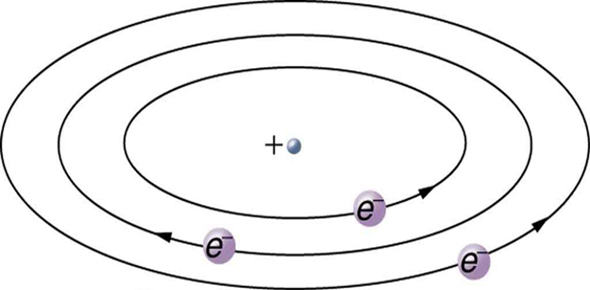Gibilisco - Electron Tubes

20 Questions | Total Attempts: 151Settings• 1.
A major difference between a triode tube and an N-channel FET is the fact that
• A.

Triodes work with lower signal voltages.

• B.

Triodes are more compact.

• C.

Triodes need higher power-supply voltages.

• D.

Triodes don’t need filaments.

• 2.
The control grid of a vacuum tube is the electrical counterpart of the
• A.

Source of a MOSFET.

• B.

Collector of a bipolar transistor.

• C.

Anode of a diode.

• D.

Gate of an FET.

• 3.
In a tetrode tube, the charge carriers are
• A.

Free electrons, which pass among the electrodes.

• B.

Holes, which are conducted within the electrodes.

• C.

Holes or electrons, depending on whether the device is P type or N type.

• D.

Nuclei of whatever elemental gas happens to exist in the tube.

• 4.
Which factor is most significant in limiting the maximum frequency at which a tube canoperate?
• A.

The power-supply voltage

• B.

The capacitance among the electrodes

• C.

The physical size of the tube

• D.

The current passing through the filament

• 5.
In a tube with a directly heated cathode,
• A.

The filament is separate from the cathode.

• B.

The grid is connected to the filament.

• C.

The filament serves as the cathode.

• D.

There is no filament.

• 6.
In a tube with a cold cathode,
• A.

The filament is separate from the cathode.

• B.

The grid is connected to the filament.

• C.

The filament serves as the cathode.

• D.

There is no filament.

• 7.
A screen grid enhances tube operation by
• A.

Increasing the gain, helping the circuit to oscillate more easily.

• B.

Decreasing the plate voltage required to produce oscillation.

• C.

Minimizing the risk that a tube amplifier will break into oscillation.

• D.

Pulling excess electrons from the plate.

• 8.
A tube with three grids is called a
• A.

Triode.

• B.

Tetrode.

• C.

Pentode.

• D.

Hexode.

• 9.
A tube with four grids is called a
• A.

Triode.

• B.

Tetrode.

• C.

Pentode.

• D.

Hexode.

• 10.
An advantage of a grounded-grid RF power amplifier over a grounded-cathode RF poweramplifier is the fact that the grounded-grid circuit
• A.

Has excellent sensitivity.

• B.

Exhibits high input impedance.

• C.

Produces little or no noise in the input.

• D.

Is more stable.

• 11.
A heptode tube has
• A.

One plate.

• B.

Two plates.

• C.

Three plates.

• D.

Four plates.

• 12.
The electron gun in a CRT is another name for its
• A.

Cathode.

• B.

Anode.

• C.

Control grid.

• D.

Screen grid.

• 13.
The electron beam in an electrostatic CRT is bent by
• A.

Magnetic fields produced by current-carrying coils.

• B.

Electric fields produced by charged electrodes.

• C.

A variable voltage on the screen grid.

• D.

Visible light striking the electrodes.

• 14.
A grounded-grid RF power amplifier
• A.

Requires more driving power, for a given RF power output, than a grounded-cathode RF power amplifier.

• B.

Requires less driving power, for a given RF power output, than a grounded-cathode RF power amplifier.

• C.

Oscillates at a more stable frequency than a grounded-cathode RF power amplifier.

• D.

Oscillates at a less stable frequency than a grounded-cathode RF power amplifier.

• 15.
In a Klystron, the electron-beam density varies as a result of
• A.

Amplitude modulation.

• B.

Pulse modulation.

• C.

Velocity modulation.

• D.

Frequency modulation.

• 16.
A vidicon camera tube is noted for its
• A.

Poor signal-to-noise ratio.

• B.

Large size and heavy weight.

• C.

Slow response to image movement in dim light.

• D.

Excellent selectivity and electrical ruggedness.

• 17.
The plate in a tetrode tube is normally connected to
• A.

A positive dc power-supply voltage.

• B.

A negative dc power-supply voltage.

• C.

Electrical ground.

• D.

RF ground.

• 18.
The screen grid in a tetrode tube is normally connected to
• A.

A positive dc power-supply voltage.

• B.

A negative dc power-supply voltage.

• C.

Electrical ground.

• D.

RF ground.

• 19.
Which of the following is most suitable for measuring the intensity of dim light?
• A.

A triode gas-filled tube

• B.

A photomultiplier tube

• C.

An electrostatic CRT

• D.

An electromagnetic CRT

• 20.
In a dissector tube, the aperture size is directly related to the
• A.

Operating voltage.

• B.

Signal-to-noise ratio.

• C.

Response speed.

• D.

Image resolution.

Related TopicsBack to top